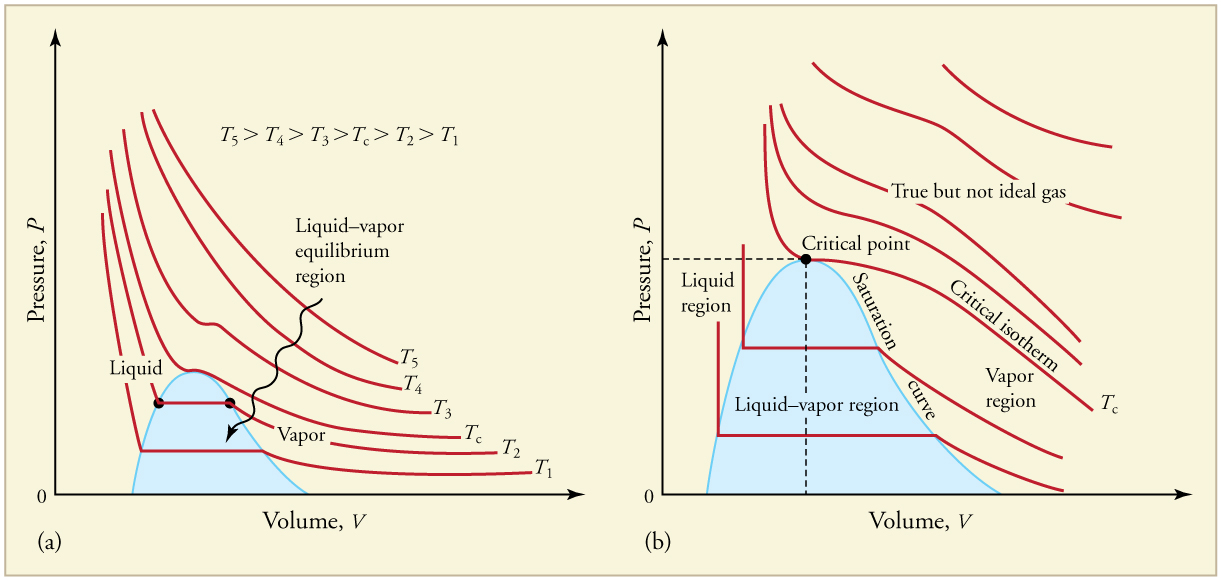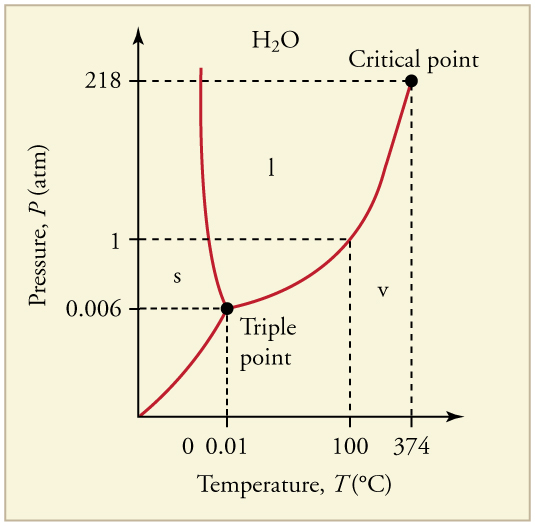# 13.5 Phase changes  (Page 2/15)

 Page 2 / 15PV size 12{ ital "PV"} {} diagrams. (a) Each curve (isotherm) represents the relationship between P size 12{P} {} and V size 12{V} {} at a fixed temperature; the upper curves are at higher temperatures. The lower curves are not hyperbolas, because the gas is no longer an ideal gas. (b) An expanded portion of the PV size 12{ ital "PV"} {} diagram for low temperatures, where the phase can change from a gas to a liquid. The term “vapor” refers to the gas phase when it exists at a temperature below the boiling temperature.
Critical temperatures and pressures
Substance Critical temperature Critical pressure
$\text{K}$ $\text{º}\text{C}$ $\text{Pa}$ $\text{atm}$
Water 647.4 374.3 $\text{22}\text{.}\text{12}×{\text{10}}^{6}$ 219.0
Sulfur dioxide 430.7 157.6 $7\text{.}\text{88}×{\text{10}}^{6}$ 78.0
Ammonia 405.5 132.4 $\text{11}\text{.}\text{28}×{\text{10}}^{6}$ 111.7
Carbon dioxide 304.2 31.1 $7\text{.}\text{39}×{\text{10}}^{6}$ 73.2
Oxygen 154.8 −118.4 $5\text{.}\text{08}×{\text{10}}^{6}$ 50.3
Nitrogen 126.2 −146.9 $3\text{.}\text{39}×{\text{10}}^{6}$ 33.6
Hydrogen 33.3 −239.9 $1\text{.}\text{30}×{\text{10}}^{6}$ 12.9
Helium 5.3 −267.9 $0\text{.}\text{229}×{\text{10}}^{6}$ 2.27

## Phase diagrams

The plots of pressure versus temperatures provide considerable insight into thermal properties of substances. There are well-defined regions on these graphs that correspond to various phases of matter, so $\text{PT}$ graphs are called phase diagrams . [link] shows the phase diagram for water. Using the graph, if you know the pressure and temperature you can determine the phase of water. The solid lines—boundaries between phases—indicate temperatures and pressures at which the phases coexist (that is, they exist together in ratios, depending on pressure and temperature). For example, the boiling point of water is $\text{100}\text{º}\text{C}$ at 1.00 atm. As the pressure increases, the boiling temperature rises steadily to $\text{374}\text{º}\text{C}$ at a pressure of 218 atm. A pressure cooker (or even a covered pot) will cook food faster because the water can exist as a liquid at temperatures greater than $\text{100}\text{º}\text{C}$ without all boiling away. The curve ends at a point called the critical point , because at higher temperatures the liquid phase does not exist at any pressure. The critical point occurs at the critical temperature, as you can see for water from [link] . The critical temperature for oxygen is $–\text{118}\text{º}\text{C}$ , so oxygen cannot be liquefied above this temperature.The phase diagram ( PT size 12{ ital "PT"} {} graph) for water. Note that the axes are nonlinear and the graph is not to scale. This graph is simplified—there are several other exotic phases of ice at higher pressures.

Similarly, the curve between the solid and liquid regions in [link] gives the melting temperature at various pressures. For example, the melting point is $0\text{º}\text{C}$ at 1.00 atm, as expected. Note that, at a fixed temperature, you can change the phase from solid (ice) to liquid (water) by increasing the pressure. Ice melts from pressure in the hands of a snowball maker. From the phase diagram, we can also say that the melting temperature of ice rises with increased pressure. When a car is driven over snow, the increased pressure from the tires melts the snowflakes; afterwards the water refreezes and forms an ice layer.

At sufficiently low pressures there is no liquid phase, but the substance can exist as either gas or solid. For water, there is no liquid phase at pressures below 0.00600 atm. The phase change from solid to gas is called sublimation    . It accounts for large losses of snow pack that never make it into a river, the routine automatic defrosting of a freezer, and the freeze-drying process applied to many foods. Carbon dioxide, on the other hand, sublimates at standard atmospheric pressure of 1 atm. (The solid form of ${\text{CO}}_{2}$ is known as dry ice because it does not melt. Instead, it moves directly from the solid to the gas state.)

full meaning of GPS system
how to prove that Newton's law of universal gravitation F = GmM ______ R²
sir dose it apply to the human system
prove that the centrimental force Fc= M1V² _________ r
prove that centripetal force Fc = MV² ______ r
Kaka
how lesers can transmit information
griffts bridge derivative
below me
please explain; when a glass rod is rubbed with silk, it becomes positive and the silk becomes negative- yet both attracts dust. does dust have third types of charge that is attracted to both positive and negative
what is a conductor
Timothy
hello
Timothy
below me
why below you
Timothy
no....I said below me ...... nothing below .....ok?
dust particles contains both positive and negative charge particles
Mbutene
corona charge can verify
Stephen
when pressure increases the temperature remain what?
what is frequency
define precision briefly
CT scanners do not detect details smaller than about 0.5 mm. Is this limitation due to the wavelength of x rays? Explain.
hope this helps
what's critical angle
The Critical Angle Derivation So the critical angle is defined as the angle of incidence that provides an angle of refraction of 90-degrees. Make particular note that the critical angle is an angle of incidence value. For the water-air boundary, the critical angle is 48.6-degrees.
okay whatever
Chidalu
pls who can give the definition of relative density?
Temiloluwa
the ratio of the density of a substance to the density of a standard, usually water for a liquid or solid, and air for a gas.
Chidalu
What is momentum
mass ×velocity
Chidalu
it is the product of mass ×velocity of an object
Chidalu
how do I highlight a sentence]p? I select the sentence but get options like copy or web search but no highlight. tks. src
then you can edit your work anyway you want
Wat is the relationship between Instataneous velocity
Instantaneous velocity is defined as the rate of change of position for a time interval which is almost equal to zero
AstronomyByByBy OpenStaxBy RhodesBy OpenStaxBy Anonymous UserBy Brooke DelaneyBy Nicole BartelsBy OpenStaxBy RhodesBy Keyaira BraxtonBy Madison Christian